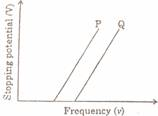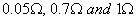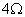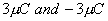Monday 01st June 2020
 CBSE Guess > Papers > Question Papers > Class XII > 2005 > Physics > Compartment Outside Delhi Set-II PHYSICS 2005 (Set II—Compartment Outside Delhi)
Except for the following questions, all the remaining questions have been asked in Set I. Q. 1. What is the value of the horizontal component of the earth's magnetic field at magnetic poles? 1 Q. 2. An electron moving through a magnetic field does not experience any force. Under what condition is this possible? 1 Q. 4. Name two factors on which electrical conductivity of a pure semiconductor at a given temperature depends. 1 Q. 6. Two signals A and B shown in the given figure are used as two inputs of a NAND gate. Draw its output wave form. Give the logic symbol of NAND gate. 2 Q. 7. With the help of a circuit diagram, explain the use of a-p-n junction diode as a full wave rectifier. 2 Q. 9. Explain the principle on which the metal detector used at airports for security reasons works. Q. 10. In a photoelectric effect experiment, the graph between the stopping potential 'V' and frequency 'v' of the incident radiations on two different metal plates P and Q are shown in the figure.(a) Which of the two metal plates, P and Q has greater value of work function?(b) What does the slope of the lines depict? 2 Q. 13. What is a laser? Explain the terms (i) stimulated emission of radiations, and (ii) population inversion. 3 Q. 14. Differentiate between pulse amplitude modulation (PAM) and pulse duration modulation (PDM). Why, is pulse code modulation (PCM) used for multi-chanel telephone communication? 3 Q. 23. Three cells of emf 2.0 V, 1.8 V and 1.5 V are connected in series. Their inter- nal resistance are 0.05respectively. If this battery is connected to an external resistor of, calculate. (a) the total current flowing in the circuit. (b) the potential difference across the terminals of the cell of emf 1.5 V while in use. 3 Q. 24. Two point chargesare located 20 cm apart in vacuum. (a) Calculate the electric field at the mid point 0 of the line AB joining the two charges. (b) What is the force experienced by a negative test charge of magnitude 1.5 x 10-9 C placed at this point? 3 Physics 2005 Question Papers Class XII Delhi Outside Delhi Compartment Delhi Compartment Outside Delhi Set I Set I Set I Set I Set II Set II Set II Set II Set III Set III Set III CBSE 2005 Question Papers Class XII